# Scatterplot in R: How to Create Scatterplot in R

Graphs and charts are visual representations of the data. If you are working in the data science field, your goal is to make sense of the large data. The data analysis contains three processes.

1. Data Extraction
2. Cleaning and manipulating the data
3. Create a graph or chart of the gathered data to analyze further.

Graphs and charts are incredible tools to simplify complex analysis. But before we begin, let’s understand some basic plotting concepts like scatter plot and correlation.

## What is a Correlation in R?

The Correlation in R is a statistical measure that shows how two variables are linearly related. In simple meaning, they change together at a constant rate.

### Positive correlation

When the y variable increases as the x variable increases, it is called a positive correlation between the variables.

### Negative correlation

When the y variable decreases as the x variable increases, it is called a negative correlation between the variables.

### No correlation

When there is no clear relationship between the two variables, there is no correlation between the two variables.

## Scatterplot in R

A Scatterplot in R is a type of data visualization that explains the relationship between two numerical variables. A scatterplot pairs up values of two quantitative variables in a data set and displays them as geometric points inside a Cartesian diagram.

A scatterplot is a set of dotted points representing individual pieces of data on the horizontal and vertical axis.

To create a scatterplot, use the plot() function. Each dataset element gets plotted as a point whose (x, y) coordinates relate to its values for the two variables.

For a data set, we will use the shows_data.csv file. From that csv file, we will use Year and IMDb columns to draw a scatterplot.

To read a csv data in R, use the read.csv() function.

``````data <- read.csv("shows_data.csv")
print(df)``````

#### Output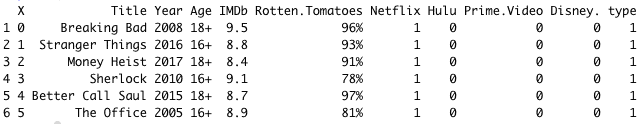We will pluck the Year and IMDb columns to create a scatter plot.

Let’s create a scatterplot of 30 rows.

``````data <- read.csv("shows_data.csv")
df <- head(data, 30)
print(df)

x <- df\$Year
y <- df\$IMDb

plot(x, y, main = "IMDB vs Year",
xlab = "Year", ylab = "IMDb Ratings",
pch = 19)``````

#### Output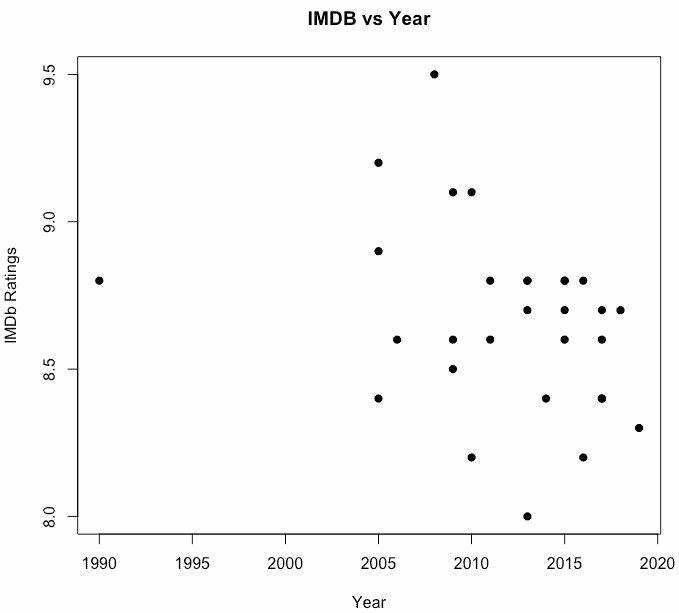Woohoo, we have successfully created a scatterplot using the plot() function.

## Use a built-in R dataset to create a scatterplot.

R provides many inbuilt datasets, and we will use the faithful dataset.

``````df <- head(faithful)
print(df)``````

#### Output

``````   eruptions  waiting
1   3.600       79
2   1.800       54
3   3.333       74
4   2.283       62
5   4.533       85
6   2.883       55``````

In the dataset faithful, we pair up the eruptions and waiting values in the same observation as (x, y) coordinates. Then we plot the points in the Cartesian plane.

``````df <- head(faithful)
print(df)

duration <- faithful\$eruptions
waiting <- faithful\$waiting

plot(duration, waiting,
xlab = "Eruption duration",
ylab = "Time waited",
main = "Duration vs Time waited"
)``````

#### Output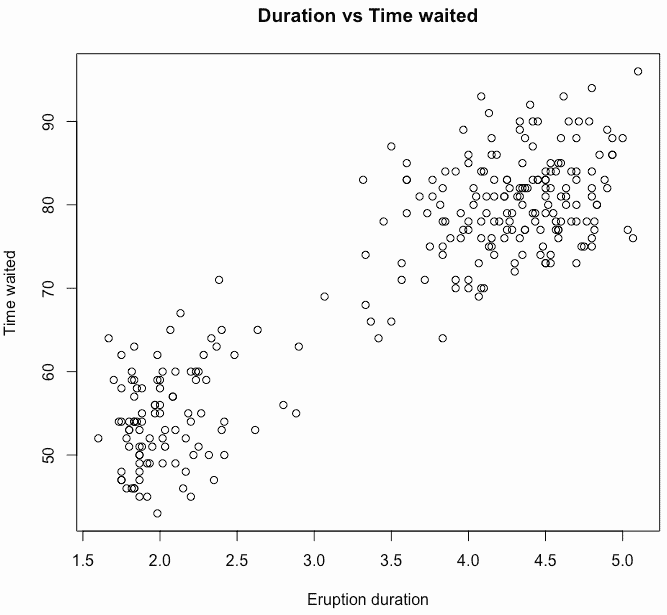## Enhanced Solution

We can generate a linear regression model of the two variables with the lm function and then draw a trend line with abline.

``abline(lm(waiting ~ duration))``

Now, see the below complete code.

``````df <- head(faithful)
print(df)

duration <- faithful\$eruptions
waiting <- faithful\$waiting

plot(duration, waiting,
xlab = "Eruption duration",
ylab = "Time waited",
main = "Duration vs Time waited"
)

abline(lm(waiting ~ duration))``````

#### Output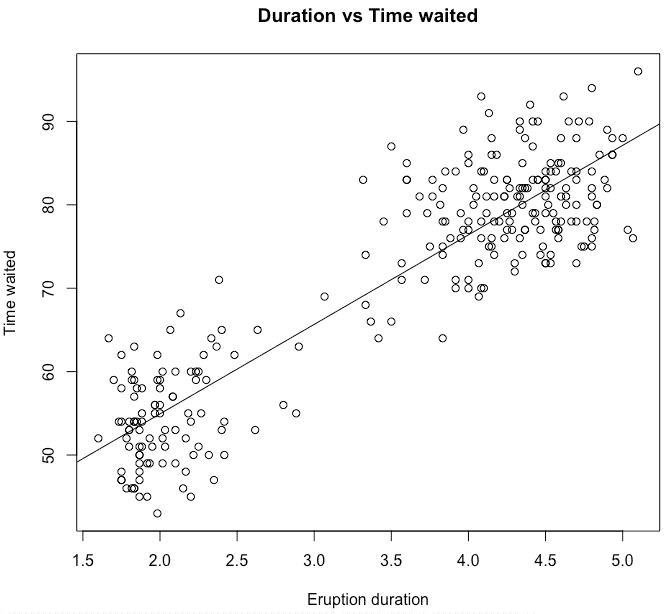## Scatterplot Matrices in R

When we have more than two variables, and we want to find the correlation between one variable versus the remaining ones, we use a scatterplot matrix. We use the pairs() function to create matrices of scatterplots.

### Syntax

``pairs(formula, data)``

### Parameters

formula: It represents the series of variables used in pairs.

data: It represents the data set from which the variables will be taken.

### Example

Each variable is paired up with each of the remaining variables. Finally, a scatterplot is plotted for each pair.

``````df <- head(mtcars)
print(df)

pairs(~wt + mpg + disp + cyl, data = mtcars,
main = "Scatterplot Matrix")``````

#### Output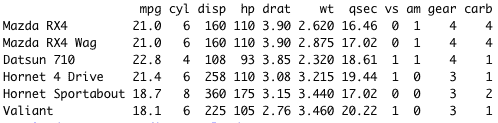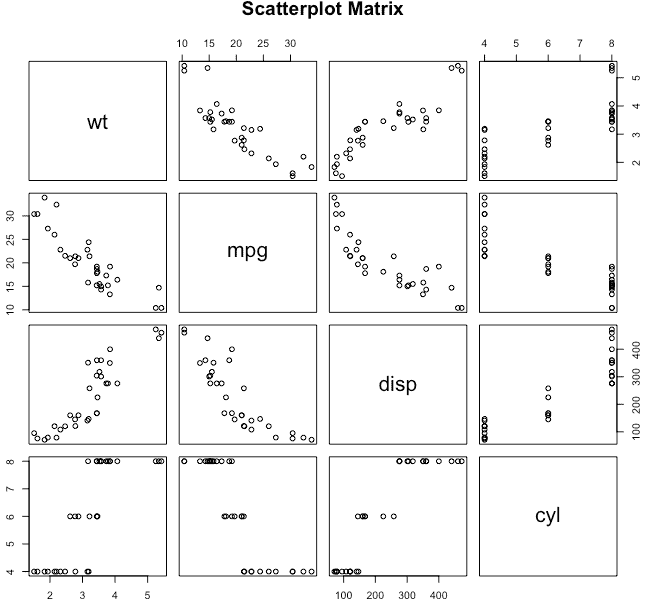And we got the scatterplots for matrices.

## High-Density scatterplot in r

If there are so many data points and significant overlap between different data points, scatter plots become less useful. To bivariate binning into hexagonal cells in R, use the hexbin() function from the hexbin package. To use the hexbin() function, you must install the hexbin package.

``````library(hexbin)

a <- rnorm(5000)
b <- rnorm(5000)
bin <- hexbin(a, b, xbins=100)
plot(bin, main="Hexagonal Binning Example")``````

#### Output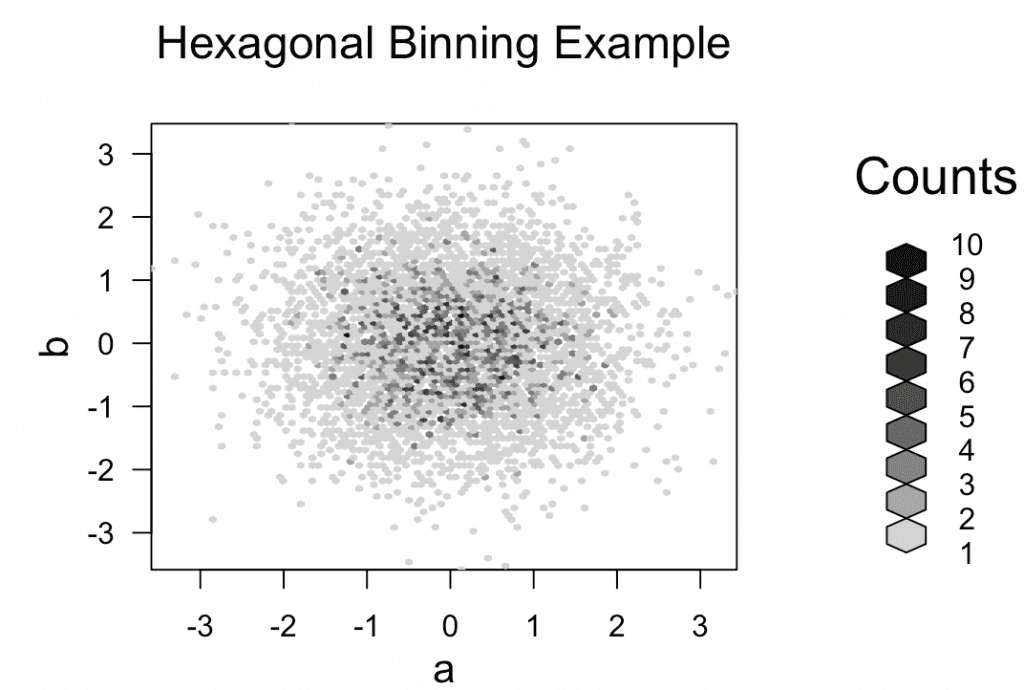To create a normal distribution of data in R, use the rnorm() function.

In this example, you can see that in the specific area of the plot, if the hexagonal count is 10, then it is filled with black color which means that area of the plot has many data points which overlap each other.

In a plot, if the hexagonal count is 1, then it is filled with gray, which means it is less crowded and does not overlap each other. To represent all the overlapped data points in the chart, we used the plot() function.

## 3D Scatterplots in R

To create a scatter plot in R, use the scatterplot3d() function from the scatterplot3d package.

For this example, we will use the built-in ChickWeight dataset.

``````library(scatterplot3d)
attach(ChickWeight)

scatterplot3d(Time, Diet, weight,
highlight.3d = TRUE,
type = "h", main = "3D Scatterplot Example"
)``````

#### Output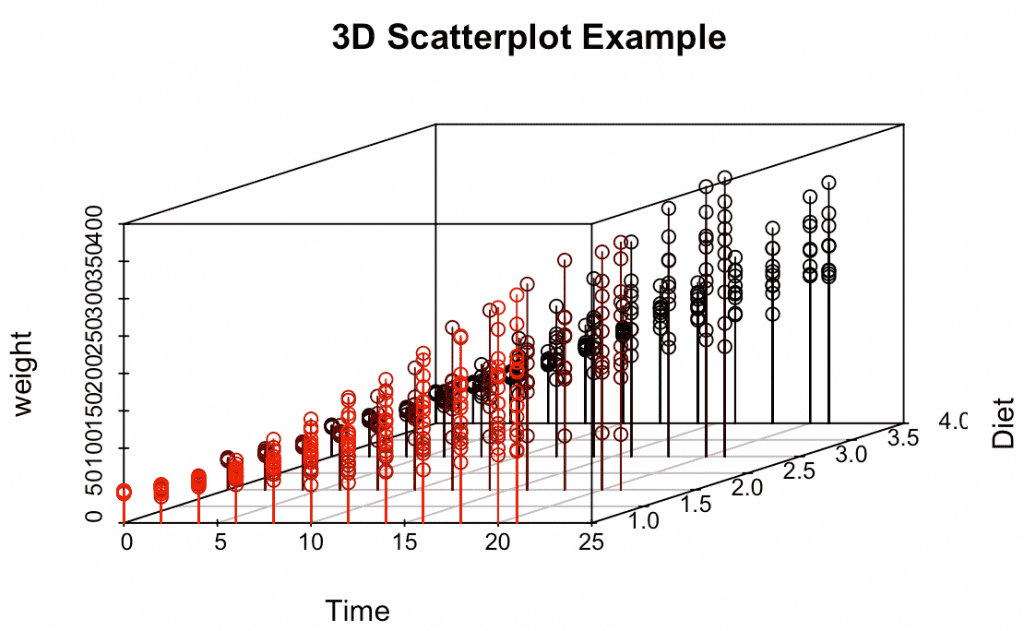As you can see that we have created a 3D scatter plot on the ChickWeight dataset.

That is it for the scatterplot in R.

## Related posts

pch in R

plot function in R

lwd in R

barchart in R

bty in R